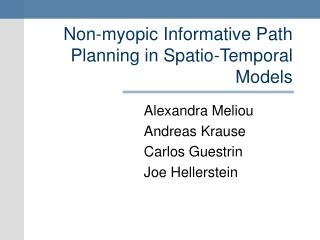DownloadDownload PresentationNon-myopic Informative Path Planning in Spatio-Temporal Models

# Non-myopic Informative Path Planning in Spatio-Temporal Models

Download Presentation## Non-myopic Informative Path Planning in Spatio-Temporal Models

- - - - - - - - - - - - - - - - - - - - - - - - - - - E N D - - - - - - - - - - - - - - - - - - - - - - - - - - -
##### Presentation Transcript

1. Non-myopic Informative Path Planning in Spatio-Temporal Models Alexandra Meliou Andreas Krause Carlos Guestrin Joe Hellerstein

2. Collection Tours

3. Approximate Queries • Approximate representation of the world: • Discrete locations • Lossy communication • Noisy measurements • Applications do not expect accurate values (tolerance to noise) • Monitored phenomena usually demonstrate strong correlations • Correlation makes approximation cheap • Example: • Return the temperature at all locations ±1C, with 95% confidence

4. Optimizing Information Search for most informative paths : sensing nodes on path Approximate answers

5. Continuous Queries • Repeated at periodic intervals • Finite horizon • Example: • Return the temperature at all locations ±1C, with 95% confidence, every 10 minutes for the next 5 hours.

6. Myopic vs Nonmyopic tradeoff Myopic approach: repeat optimization for every timestep Timestep 1 Timestep 2

7. Myopic vs Nonmyopic tradeoff Nonmyopic approach: optimize for all timesteps No work! Timestep 1 Timestep 2 Extra node

8. Quantify Informativeness • Entropy • [Shewry & Wynn ‘87] • Mutual Information • [Caselton & Zidek ‘84] • Reduction of predictive variance • [Chaloner & Verdinelli ‘95]

9. Measuring Information Observing 1 gives information on 3 and 4 Observing 2 gives information on 3 and 5 1 2 3 4 5 After observing 2, observing 3 becomes less useful Diminishing Returns

10. B A Submodular Functions X More reward + X + Less reward Entropy, mutual information and reduction of predictive variance are all submodular.

11. Non-myopic Spatio-Temporal Path Planning (NSTP) • Given: • A collection of submodular functions ft • ftonly depends on data collected at times 1..t • A set of accuracy constraints kt • Find: • A collection of paths Ptwith Minimize cost Subject to reward constraints

12. Planning for multiple timesteps obviously • Harder than planning for one • First idea : • Solve an equivalent single step problem instead!

13. Nonmyopic Planning Graph t=1 t=2 t=3 A solution path on the NPG = collection of paths for multiple timesteps

14. Solve the single step problem • NP hard • No good known approximation guarantees • Dual: Submodular Orienteering Problem Maximize reward Minimize cost dual: primal: Subject to budget constraints Subject to reward constraints

15. Good News • The dual algorithm [Chekuri & Pal ’05] provides an O(logn) factor approximation (where n is the size of the network)

16. Covering Algorithm • Transform a dual blackbox solution to a primal solution dual: Call with BOPT Return solution with reward ≥K/α (with α approximation factor) primal: Reward required to “cover”

17. CoveringAlgorithm • Transform a dual blackbox solution to a primal solution • Call SOP for increasing budgets uncovered reward • Call for budget 1 • Call for budget 2 • Call for budget BOPT : insufficient reward : reward sufficient! • Guaranteed to cover K/α reward when called for BOPT • Update chosen set and repeat for uncovered reward • Terminate when ε portion left Reward required to “cover” Guaranteed to use at most budget

18. Bad News • On the unrolled graph the Chekuri-Pal guarantee becomes O(log(nT)) • The running time on the unrolled graph is O((BnT)log(nT))

19. Addressing Computation Complexity • DP Algorithm • Algorithm details in proceedings • Bug in proof of guarantees. Not fixed (yet) • New algorithm: Nonmyopic Greedy • Details on my webpage… • Guaranteed to provide O(logn) approximation Better than the previous O(log(nT))

20. Approach • Replace expensive blackbox, with cheaper blackbox More efficient: Nonmyopic greedy calls the dual on the smaller network graph instead of the unrolled graph Covering transformation Blackbox for dual Blackbox for dual Nonmyopic greedy algorithm Chekuri-Pal SOP on NPG

21. Nonmyopic Greedy 1. Condition on picked data 2. Recompute matrix Time Return best of A1, A2 Best ratio R/C R = 0 C = 1 R = 2 C = 1 R = 2 C = 1 R = 1 C = 1 R = 0 C = 1 R = 1 C = 1 R = 1 C = 1 R = 1 C = 1 R = 1 C = 1 budget dual(budget=1,time=3) Budget P3 Cost = 1 Time = 3 R = 3 C = 2 X R = 2 C = 2 X R = 1 C = 2 R = 4 C = 2 X R = 1 C = 2 R = 3 C = 2 For border cases were A1 is bad, A2 is guaranteed to be good P2 Cost = 1 Time = 1 X R = 3 C = 2 R = 5 C = 3 X R = 4 C = 3 X A1 dual(b,Gt) P1 Cost = 2 Time = 2 A2 R = 5 C = 4 X R = 6 C = 4 X X R = 5 C = 4 Best greedy choice condition on A1 dual(budget=4,time=1)

22. Nonmyopic Greedy Guarantees running time approximation

23. Myopic and Nonmyopic evaluation • Setup: • 46 nodes on the Intel Berkeley Lab deployment • 7 days of data (5 for learning, 2 for testing) Varying Constraints

24. Cost and Runtime Varying Horizon

25. Effect of greedy parameters Varying budget levels

26. Conclusions • Transform any blackbox solution to nonmyopic • Obtain primal from dual • Nonmyopic greedy provides significant runtime improvements and better theoretical guarantees